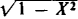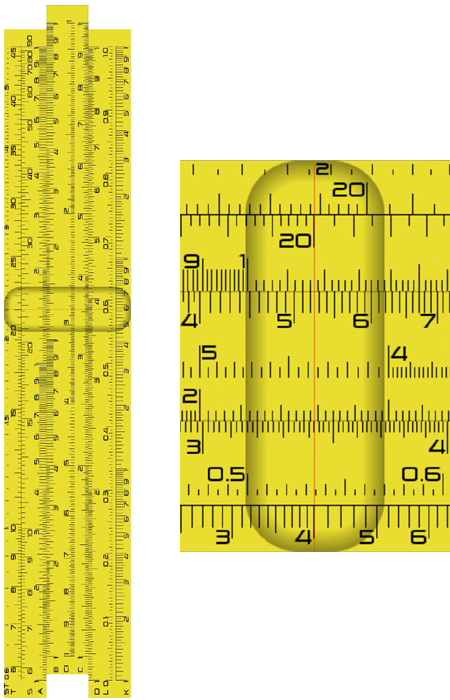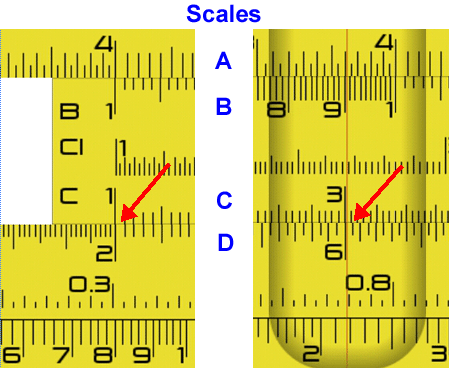# slide rule

(redirected from Slipsticks)
Also found in: Dictionary, Thesaurus, Idioms.

## slide rule,

instrument for making numerical computations and readings, the results of which may be read easily and quickly after performing simple mechanical manipulations. Multiplication and division, finding of powers and roots, and other more complicated calculations may be performed with a slide rule. Based on John Napier's principle of the logarithmlogarithm
[Gr.,=relation number], number associated with a positive number, being the power to which a third number, called the base, must be raised in order to obtain the given positive number.
, it came into use after Edmund Gunter created a logarithmic scale in 1620. Gunter's rule consisted of a straight line on which numbers were spaced at intervals proportional to their common logarithms. Using this scale, William Oughtred and Edmund Wingate developed independently (c.1630) the first slide rules. Amédée Mannheim, a French army officer, in 1850 established the form that it maintained thereafter. This had three parts, the stock, the slide, and the cursor (indicator). The stock consisted of two fixed parallel rules, each with a scale on its inner edge. The slide was a single rule, moving between them. It had two scales on its outer edge, each scale corresponding to the fixed scale to which it was adjacent. The cursor, a transparent square with a hairline, could be moved the length of the rule to aid in reading it. In its many varieties it had additional scales for such calculation as logarithms, trigonometric functions, and square roots. By the late 1980s they had been supplanted by the electronic calculators, which were easier to use and more accurate.
The following article is from The Great Soviet Encyclopedia (1979). It might be outdated or ideologically biased.

## Slide Rule

an instrument for simple calculations by means of which operations on numbers (multiplication, division, raising to a power, extraction of a root) are replaced by operations on the logarithms of these numbers.

A slide rule consists of a body, a slide, and a cursor, or indicator (made of glass or plexiglass), with a hairline. The basic C and D scales are marked on the body and slide. The scales are graduated such that the position of any number X (integral or fractional from 1 to 10) is determined by the length of a segment that is equal to μ log X (μ is the scale factor, or modulus of the scale) and laid off from the beginning of the scale. The geometric addition (subtraction) of segments of the C and D scales by displacing the slide with respect to the body on the slide rule replaces the operation of multiplication of the corresponding numbers. In addition to the C and D scales, many slide rules also carry the R scale (for computing 1/X), the A and B scales (for computing X2) the K scale (for computing X3), the L scale (for computing log X), scales for computingand ex, and scales of the values of the trigonometric functions, among others.

The slide rule, whose prototype was the Gunter’s line, was invented by the British mathematician E. Gunter soon after the discovery of logarithms and was described by him in 1623. The device consisted of a logarithmic scale (rule) on which addition of segments was performed by means of dividers. In 1630 the British mathematician W. Oughtred replaced the dividers with a second rule (the slide). Various improvements of the original design were made over the succeeding years. In 1650 the scales were laid along a helical line on a cylindrical surface. The 1830’s saw the appearance of a device that followed the principle of Gunter’s line but was made in the form of a clock with a rotating dial (logarithmic scale) with a movable pointer, the prototype of modern logarithmic circular slide rules. A cursor, or indicator, was added to the slide rule in 1850, which significantly simplified its operation. A cylindrical slide rule, a type of slide rule whose scales were laid off on the elements of the cylindrical rollers, was developed in the early 20th century to provide increased accuracy in calculations. A hollow cylinder with windows notched opposite the basic scales served as the slide, whose scale was marked along the edges of the notches.

The modern slide rule is a simple and convenient computational tool. It is used in engineering and other calculations requiring an accuracy to within two or three places (for an ordinary slide rule 25 cm in length with μ = 250 mm). Slide rules with μ = 500 to 750 mm are accurate to within four or five places.

### REFERENCE

Panov, D. Iu. Schetnaia lineika, 21st ed. Moscow, 1973.

## slide rule

[′slīd ‚rül]
(mathematics)
A mechanical device, composed of a ruler with sliding insert, marked with various number scales, which facilitates such calculations as division, multiplication, finding roots, and finding logarithms.
McGraw-Hill Dictionary of Scientific & Technical Terms, 6E, Copyright © 2003 by The McGraw-Hill Companies, Inc.

## slide rule

Obsolete
a mechanical calculating device consisting of two strips, one sliding along a central groove in the other, each strip graduated in two or more logarithmic scales of numbers, trigonometric functions, etc. It employs the same principles as logarithm tables
Collins Discovery Encyclopedia, 1st edition © HarperCollins Publishers 2005

## slide rule

An early computing device invented by Reverend William Oughtred in London in the 17th century. Primarily for multiplication and division, the slide rule has two stationary sets and one sliding set of numbers in the center. To multiply, numbers are added; to divide, numbers are subtracted. Subsequent slide rules added scales for trigonometric, powers and root computations.

Slide Rule and CursorThe vertical hairline on the movable "cursor" enables precise viewing. Because the slide rule is based on a logarithmic scale, the spacing between numerical divisions decreases as the values increase. These examples were taken from the Android Slide Rule app from Gushiku Studios LLC.

Multiply 2 Times 3The 1 on the C scale is set to 2 on the D scale. Then the cursor is moved to 3 on the C scale, and the result is read on the D scale.
Copyright © 1981-2019 by The Computer Language Company Inc. All Rights reserved. THIS DEFINITION IS FOR PERSONAL USE ONLY. All other reproduction is strictly prohibited without permission from the publisher.
Site: Follow: Share:
Open / Close